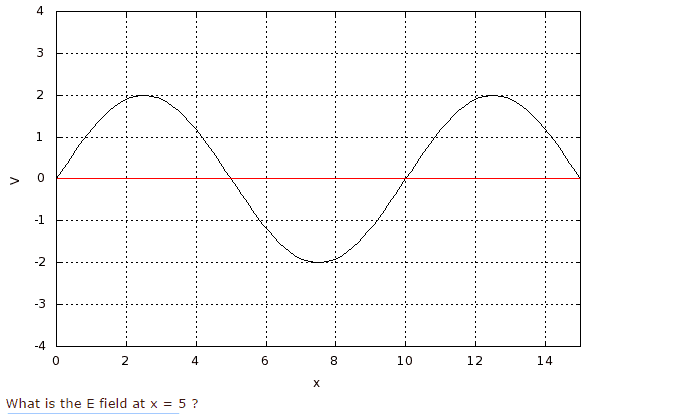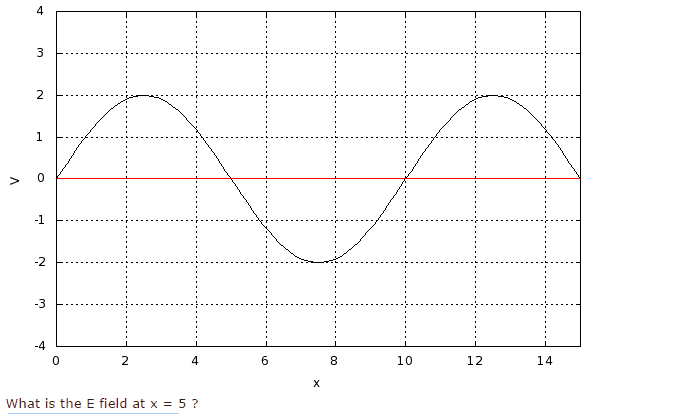# E field at point from graph of V and x?

## Homework StatementE = -dV/dx ?

## The Attempt at a Solution

not really sure how to do this problem. I think I understand E field is negative of slope of V/x, but i'm getting it wrong. just looking at it, I see V=-2 at x=7.5. so the E field to right of x= 5 is y2-y1/x2-x1= 2/2.5 = 0.8. to the left of it I guess it's -0.8 (V/m). I tried both answer but its wrong.

collinsmark
Homework Helper
Gold Member

## Homework StatementE = -dV/dx ?

## The Attempt at a Solution

not really sure how to do this problem. I think I understand E field is negative of slope of V/x, but i'm getting it wrong. just looking at it, I see V=-2 at x=7.5. so the E field to right of x= 5 is y2-y1/x2-x1= 2/2.5 = 0.8. to the left of it I guess it's -0.8 (V/m). I tried both answer but its wrong.

Finding the slope is the correct approach. So in that respect you are on the right track.

But the way in which you are going about finding the slope is too inaccurate. There's a better way.

Can you express V(x) in terms of a sine function? In other words, if

V(x) = A sin(kx),

can you find A and k ?

oh yeah, I didn't think of that. V(x) = 2*sin(0.2*pi*x), so I guess I take the derivative of this to find E field? dV/dx = 2*0.2*pi*cos(0.2*pi*x), and at x=5, E=-dV/dx=1.26?

collinsmark
Homework Helper
Gold Member
oh yeah, I didn't think of that. V(x) = 2*sin(0.2*pi*x), so I guess I take the derivative of this to find E field? dV/dx = 2*0.2*pi*cos(0.2*pi*x), and at x=5, E=-dV/dx=1.26?

That looks correct to me! :)

(Don't forget to include the proper units in the final answer through. [Edit: then again, the problem statement didn't specify the units of V nor x. So nevermind about that, I guess.])

Last edited: